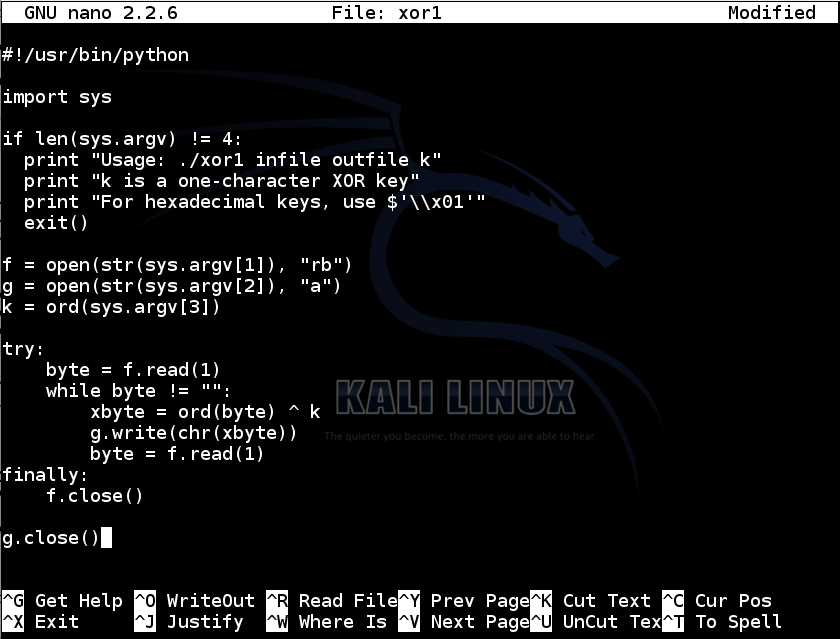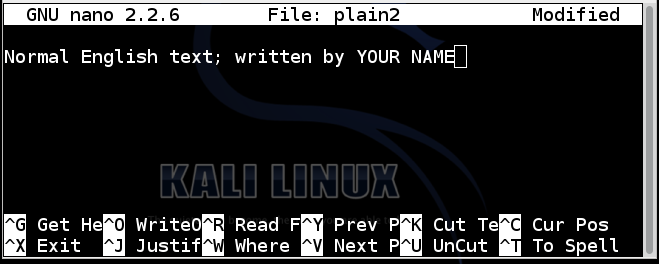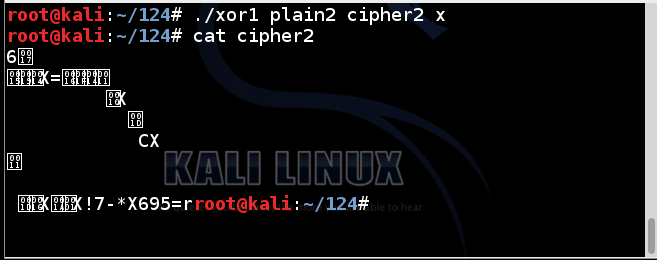# XOR Encryption in Python

## What You Need

A Kali Linux machine, real or virtual. You could also use OS X, or Windows with Python installed.

## Purpose

Encrypt and decrypt files using XOR in Python.

## Understanding XOR

Exclusive OR (XOR) is a fundamental mathematical operation used in many encryption algorithms.

XOR operates on one bit at a time, with these results:

``` 0 XOR 0 = 0 0 XOR 1 = 1 1 XOR 0 = 1 1 XOR 1 = 0 ```
For our purposes, we'll use the Python ^ operator, which acts on a whole byte at a time.

Characters are ASCII-encoded, like this:

``` A is 01000001 B is 01000010 C is 01000011 ... ```
A whole table of ASCII values is here:

Consider A^B:

``` A is 01000001 B is 01000010 A^B= 00000011 ```
That is character 3, an unprintable end-of-text mark.

However, A^s is printable:

``` A is 01000001 s is 01110011 A^B= 00110010 ```
The result is the hexadecimal value 0x32, or the numeral 2.

## XOR in Python

In Kali Linux, in a Terminal window, execute this command:
```nano xor1 ```
In nano, enter the code shown below:
```#!/usr/bin/python import sys if len(sys.argv) != 4: print "Usage: ./xor1 infile outfile k" print "k is a one-character XOR key" print "For hexadecimal keys, use \$'\\x01'" exit() f = open(str(sys.argv), "rb") g = open(str(sys.argv), "a") k = ord(sys.argv) try: byte = f.read(1) while byte != "": xbyte = ord(byte) ^ k g.write(chr(xbyte)) byte = f.read(1) finally: f.close() g.close() ```Save the file with Ctrl+X, Y, Enter. Next, we need to make the file executable.

In a Terminal window, execute this command:

```chmod a+x xor1 ```

## Encrypting a Single Character

In a Terminal window, execute this command:
```./xor1 ```
You see the help message, explaining how to use the program, as shown below.To create a file named plain1 with the letter A in it, execute these commands :

```echo -n A > plain1 cat plain1 ```
The "echo -n" command created a file named plain1 which contains a single letter A, without a carriage return at the end of the file.

The "cat plain1" command printed out the file, which appeared as a single A at the start of the next line, as shown below:To encode the plain1 file with a key of s, execute these commands:

```./xor1 plain1 cipher1 s cat cipher1 ```
The result is 2, as shown below:## Encrypting a Text File

In Kali Linux, in a Terminal window, execute this command:
```nano plain2 ```
In nano, enter the code shown below, replacing "YOUR NAME" with your own name:
```Normal English text; written by YOUR NAME ```Save the file with Ctrl+X, Y, Enter. To encrypt the file using a key of x, execute these commands:

```./xor1 plain2 cipher2 x cat cipher2 ```
The result is strange unreadable characters, as shown below:## Decrypting a Text File

To decrypt a file, simply repeat the XOR operation with the same key. It will reverse itself.

Execute these commands:

```./xor1 cipher2 plain2r x cat plain2r ```
The file is restored to readable text, as shown below:Last revised: 8-4-16